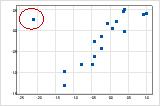# Interpret the key results for Matrix Plot

Complete the following steps to interpret a matrix plot.

## Step 1: Look for model relationships and assess the strength

Look for model relationships between pairs of variables. Determine which model relationship best fits your data and assess the strength of the relationship. If a model fits well, you can use the regression equation for that model to describe your data.

###### Tip

To see how well a particular model fits your data, add a fitted regression line. Double-click the graph. With the graph in editing mode, right-click the graph, then choose Add > Regression Fit. You can hold the pointer over the fitted regression line to see the regression equation.

### Type of relationship

The following examples show different types of relationships you can model with a regression fit line.

If your data seem to fit a model, you can explore the relationship using a regression analysis.

### Strength of relationship

Assess how closely the data fit the model to estimate the strength of the relationship between X and Y. When the relationship is strong, the regression equation models the data accurately. If you have a fitted regression line, hold the pointer over it to view the regression equation and the R-squared value. The higher the R-squared value, the more accurately the regression equation models your data.

To quantify the strength of a linear (straight) relationship, use a correlation analysis.

## Step 3: Look for other patterns

Outliers may indicate unusual conditions in your data. Time-based trends may indicate changing data conditions.

### Outliers

Outliers, which are data values that are far away from other data values, can strongly affect your results.

On a scatterplot, isolated points identify outliers.Try to identify the cause of any outliers. Correct any data entry or measurement errors. Consider removing data values that are associated with abnormal, one-time events (special causes). Then, repeat the analysis.

By using this site you agree to the use of cookies for analytics and personalized content.  Read our policy